#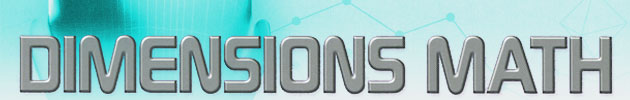Update: This review is now QUITE dated! Dimensions Math has expanded to offer PreK-6th, though at the time of this update 5th grade remains unfinished. 7th & 8th grade books have been revised to meet the "Common Core" standards and renamed Dimensions Math. The books for 9th and above, called Discovering Mathematics, have been discontinued and there are no current plans to replace them. We plan to completely overhaul this review when the series is complete.

Another option from Singapore for secondary math instruction, Discovering Mathematics will appeal especially to those looking for a largely student-directed program. The colorful worktexts present concepts clearly and completely, without being verbose or overly repetitive. Singapore is noted for its superior math instruction, and this series is a worthy addition to the already impressive selection.

## How Do These Work?

The course covers grades 7-10. For each grade there are two student textbooks and two teacher guides. The student texts are not consumable; students complete exercises on a separate sheet of paper. Each text is divided into several chapters which are further divided into digestible units. Chapters open with a brief interesting history or real-life application relating to the topic, followed by text and a number of examples.

Exercises are grouped according to purpose. After the chapter unit students complete basic practice problems which serve as the practical introduction to the topic. Further practice problems are more complicated and nuanced, and the rest of the exercises are designed to show the practical application of the topic and help kids improve their logic and analytical skills. Students are also encouraged to keep a math journal where they record real-life contexts in which various topics might be useful.

This is a fairly fast-moving course. By the final book students are doing statistics and probability and advanced trigonometry. From there, they can move on to calculus and physics for the final years of high school, though they will also be prepared to move on to most first-level college math courses.

There isn't a lot of review. Topics are taught for mastery, and thoroughly covered and practiced before moving on to the next one. Since instruction is so clear and there are so many worked examples in the text, most students who can pay attention and apply themselves shouldn't have too much trouble getting through lessons. Since the emphasis is on practical application of mathematical concepts, exercises are constructed to help students internalize information by following each step in the process carefully.

Discovering Mathematics is more or less a student-directed course. The teacher guides include a yearly lesson plan, brief teaching notes and fully worked solutions to all exercises. While the teaching notes are helpful, they aren't nearly enough to teach the course on, and are really only supplemental bits of information and suggestions for class activities and presentation. Of all the secondary Singapore math courses, this is the least teacher-reliant. As with any course, teacher involvement can't hurt, but you'll need to put together your own lesson plans and should probably have a pretty thorough knowledge of each topic you introduce.

## Our Honest Opinion:

It's hard to say anything negative about Singapore math products. Clear text, high standards of student progress and tough yet workable exercises have become the rule for their courses. Discovering Mathematics is no exception, and is even one of the best programs Singapore has to offer. If your students like math or want to pursue a math or science related career or course of study in college, this would be an excellent place to start. Even students who don't like math as much will benefit greatly from the logical presentation of advanced math concepts. Overall, this is one of the best junior high/high school math courses we offer.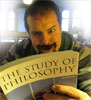Review by C. Hollis Crossman C. Hollis Crossman used to be a child. Now he is a husband and father, teaches adult Sunday school in his Presbyterian congregation, and likes weird stuff. He might be a mythical creature, but he's definitely not a centaur.Read more of his reviews here.

Did you find this review helpful?
78 Items found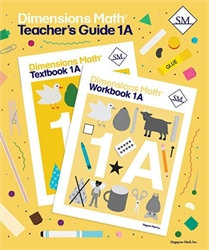Dimensions Math 1A - Teacher's Guide
from Singapore Math
in Dimensions Mathematics (Location: MAT-SMDM)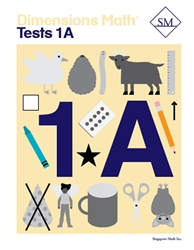Dimensions Math 1A - Tests
from Singapore Math
in Dimensions Mathematics (Location: MAT-SMDM)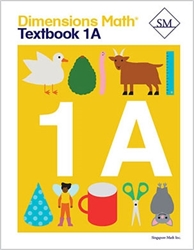Dimensions Math 1A - Textbook
from Singapore Math
in Dimensions Mathematics (Location: MAT-SMDM)
\$12.00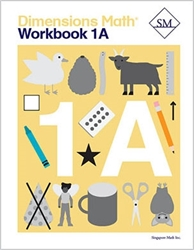Dimensions Math 1A - Workbook
from Singapore Math
in Dimensions Mathematics (Location: MAT-SMDM)
\$12.00Dimensions Math 1B - Teacher's Guide
from Singapore Math
in Dimensions Mathematics (Location: MAT-SMDM)
\$25.00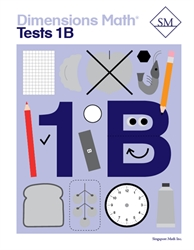Dimensions Math 1B - Tests
from Singapore Math
in Dimensions Mathematics (Location: MAT-SMDM)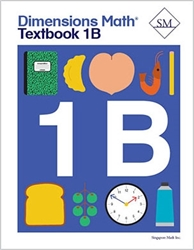Dimensions Math 1B - Textbook
from Singapore Math
in Dimensions Mathematics (Location: MAT-SMDM)
\$12.00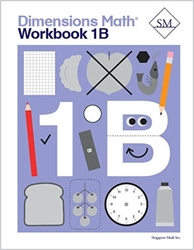Dimensions Math 1B - Workbook
from Singapore Math
in Dimensions Mathematics (Location: MAT-SMDM)
\$12.00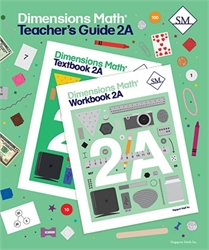Dimensions Math 2A - Teacher's Guide
from Singapore Math
in Dimensions Mathematics (Location: MAT-SMDM)
\$25.00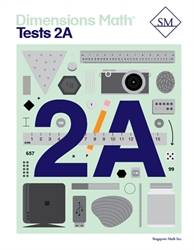Dimensions Math 2A - Tests
from Singapore Math
in Dimensions Mathematics (Location: MAT-SMDM)
\$12.00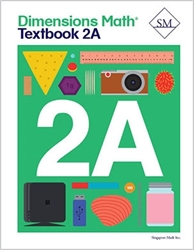Dimensions Math 2A - Textbook
from Singapore Math
in Dimensions Mathematics (Location: MAT-SMDM)
\$12.00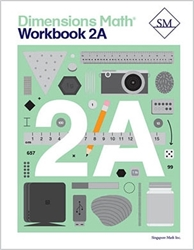Dimensions Math 2A - Workbook
from Singapore Math
in Dimensions Mathematics (Location: MAT-SMDM)
\$12.00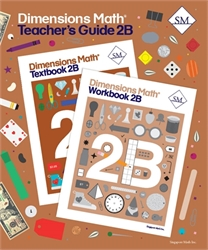Dimensions Math 2B - Teacher's Guide
from Singapore Math
in Dimensions Mathematics (Location: MAT-SMDM)
\$25.00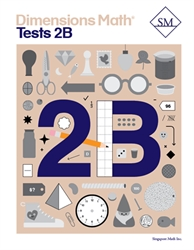Dimensions Math 2B - Tests
from Singapore Math
in Dimensions Mathematics (Location: MAT-SMDM)
\$12.00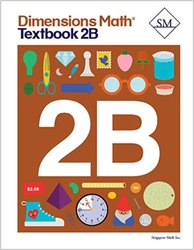Dimensions Math 2B - Textbook
from Singapore Math
in Dimensions Mathematics (Location: MAT-SMDM)
\$12.00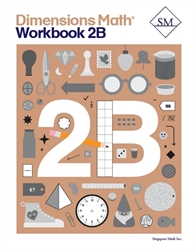Dimensions Math 2B - Workbook
from Singapore Math
in Dimensions Mathematics (Location: MAT-SMDM)
\$12.00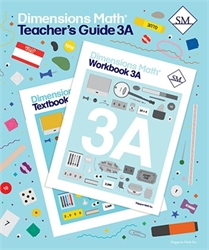Dimensions Math 3A - Teacher's Guide
from Singapore Math
in Dimensions Mathematics (Location: MAT-SMDM)
\$25.00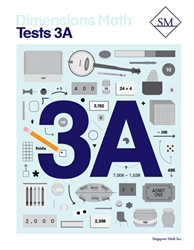Dimensions Math 3A - Tests
from Singapore Math
in Dimensions Mathematics (Location: MAT-SMDM)
\$12.00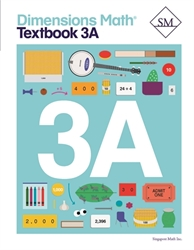Dimensions Math 3A - Textbook
from Singapore Math
in Dimensions Mathematics (Location: MAT-SMDM)
\$12.00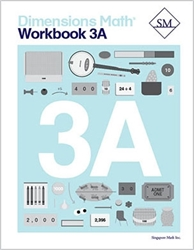Dimensions Math 3A - Workbook
from Singapore Math
in Dimensions Mathematics (Location: MAT-SMDM)
\$12.00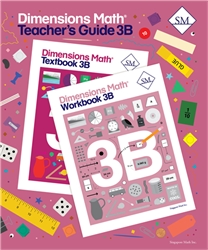Dimensions Math 3B - Teacher's Guide
from Singapore Math
in Dimensions Mathematics (Location: MAT-SMDM)
\$25.00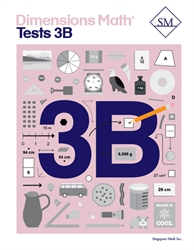Dimensions Math 3B - Tests
from Singapore Math
in Dimensions Mathematics (Location: MAT-SMDM)
\$12.00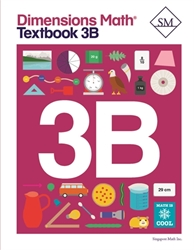Dimensions Math 3B - Textbook
from Singapore Math
in Dimensions Mathematics (Location: MAT-SMDM)
\$12.00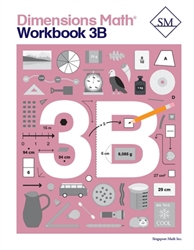Dimensions Math 3B - Workbook
from Singapore Math
in Dimensions Mathematics (Location: MAT-SMDM)
\$12.00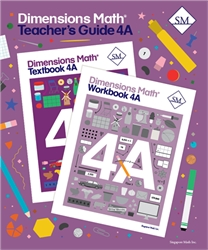Dimensions Math 4A - Teacher's Guide
from Singapore Math
in Dimensions Mathematics (Location: MAT-SMDM)
\$25.00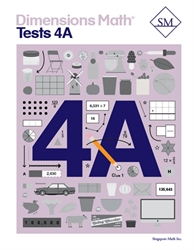Dimensions Math 4A - Tests
from Singapore Math
in Dimensions Mathematics (Location: MAT-SMDM)
\$12.00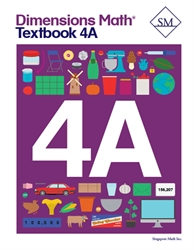Dimensions Math 4A - Textbook
from Singapore Math
in Dimensions Mathematics (Location: MAT-SMDM)
\$12.00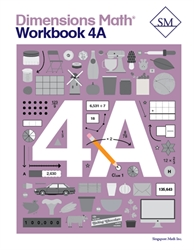Dimensions Math 4A - Workbook
from Singapore Math
in Dimensions Mathematics (Location: MAT-SMDM)
\$12.00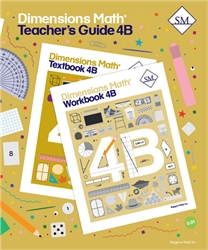Dimensions Math 4B - Teacher's Guide
from Singapore Math
in Dimensions Mathematics (Location: MAT-SMDM)
\$25.00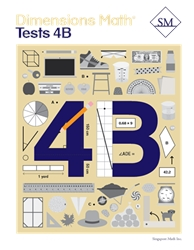Dimensions Math 4B - Tests
from Singapore Math
in Dimensions Mathematics (Location: MAT-SMDM)
\$12.00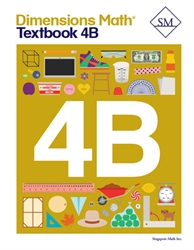Dimensions Math 4B - Textbook
from Singapore Math
in Dimensions Mathematics (Location: MAT-SMDM)
\$12.00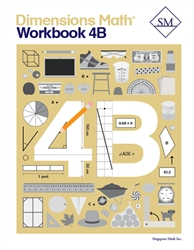Dimensions Math 4B - Workbook
from Singapore Math
in Dimensions Mathematics (Location: MAT-SMDM)
\$12.00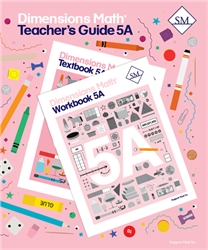Dimensions Math 5A - Teacher's Guide (2020)
from Singapore Math
in Dimensions Mathematics (Location: MAT-SMDM)
\$25.00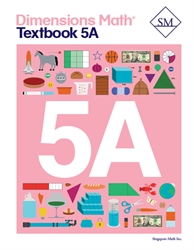Dimensions Math 5A - Textbook
from Singapore Math
in Dimensions Mathematics (Location: MAT-SMDM)
\$12.00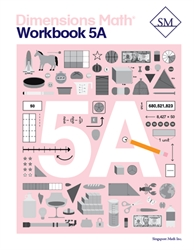Dimensions Math 5A - Workbook
from Singapore Math
in Dimensions Mathematics (Location: MAT-SMDM)
\$12.00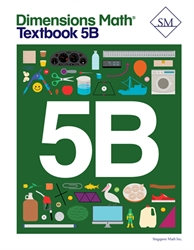Dimensions Math 5B - Textbook (June 2020)
from Singapore Math
in Dimensions Mathematics (Location: MAT-SMDM)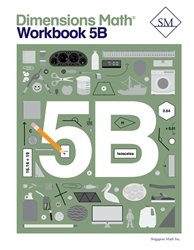Dimensions Math 5B - Workbook (July 2020)
from Singapore Math
in Dimensions Mathematics (Location: MAT-SMDM)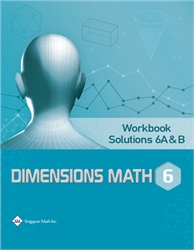Dimensions Math 6A & 6B - Workbook Solutions
from Star Publishing
in Dimensions Mathematics (Location: MAT-SMDM)
\$10.00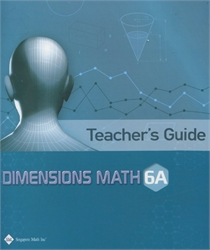Dimensions Math 6A - Teacher's Guide
from Star Publishing
in Dimensions Mathematics (Location: MAT-SMDM)
\$29.00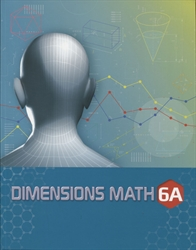Dimensions Math 6A - Textbook
from Star Publishing
in Dimensions Mathematics (Location: MAT-SMDM)
\$22.50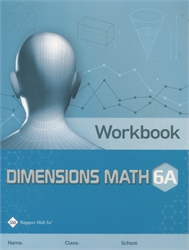Dimensions Math 6A - Workbook
from Star Publishing
in Dimensions Mathematics (Location: MAT-SMDM)
\$12.00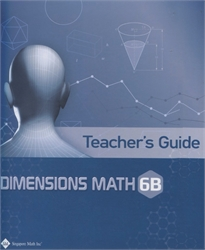Dimensions Math 6B - Teacher's Guide
from Star Publishing
in Dimensions Mathematics (Location: MAT-SMDM)
\$29.00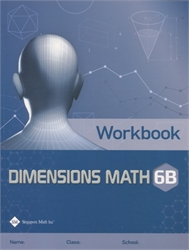Dimensions Math 6B - Workbook
from Star Publishing
in Dimensions Mathematics (Location: MAT-SMDM)
\$12.00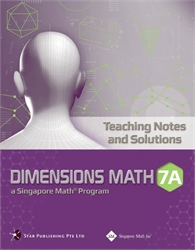Dimensions Math 7A - Teaching Notes & Solutions
from Star Publishing
in Dimensions Mathematics (Location: MAT-SMDM)
\$31.00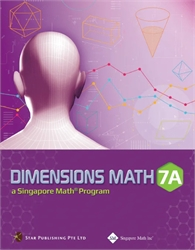Dimensions Math 7A - Textbook
from Singapore Math
in Dimensions Mathematics (Location: MAT-SMDM)
\$24.00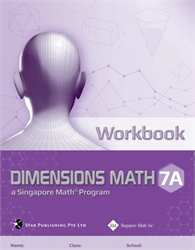Dimensions Math 7A - Workbook
from Singapore Math
in Dimensions Mathematics (Location: MAT-SMDM)
\$11.00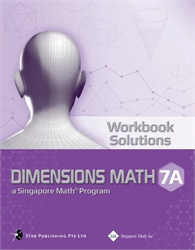Dimensions Math 7A - Workbook Solutions
from Singapore Math
in Dimensions Mathematics (Location: MAT-SMDM)
\$16.50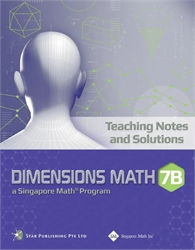Dimensions Math 7B - Teaching Notes & Solutions
from Singapore Math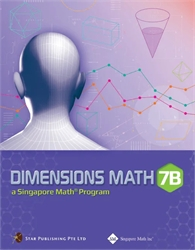Dimensions Math 7B - TextbookDimensions Math 7B - Textbook